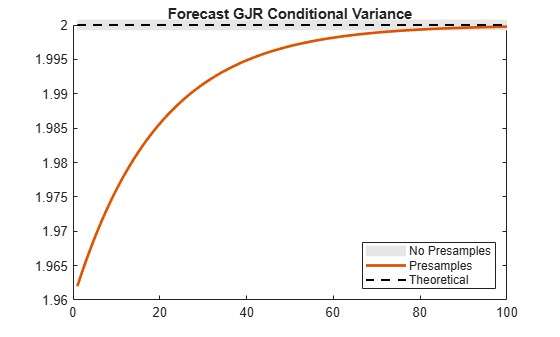# Forecast GJR Models

This example shows how to generate MMSE forecasts from a GJR model using `forecast`.

### Step 1. Specify a GJR model.

Specify a GJR(1,1) model without a mean offset and $\kappa =0.1$, ${\gamma }_{1}=0.7$, ${\alpha }_{1}=0.2$ and ${\xi }_{1}=0.1$.

```Mdl = gjr('Constant',0.1,'GARCH',0.7,... 'ARCH',0.2,'Leverage',0.1);```

### Step 2. Generate MMSE forecasts.

Generate forecasts for a 100-period horizon with and without specifying a presample innovation and conditional variance. Plot the forecasts along with the theoretical unconditional variance of the model.

```v1 = forecast(Mdl,100); v2 = forecast(Mdl,100,'Y0',1.4,'V0',2.1); denom = 1-Mdl.GARCH{1}-Mdl.ARCH{1}-0.5*Mdl.Leverage{1}; sig2 = Mdl.Constant/denom; figure plot(v1,'Color',[.9,.9,.9],'LineWidth',8) hold on plot(v2,'LineWidth',2) plot(ones(100,1)*sig2,'k--','LineWidth',1.5) xlim([0,100]) title('Forecast GJR Conditional Variance') legend('No Presamples','Presamples','Theoretical',... 'Location','SouthEast') hold off````v2(1) % Display forecasted conditional variance`
```ans = 1.9620 ```

The forecasts generated without using presample data are equal to the theoretical unconditional variance. In the absence of presample data, `forecast` uses the unconditional variance for any required presample innovations and conditional variances.

In this example, for the given presample innovation and conditional variance, the starting forecast is

`${\underset{}{\overset{ˆ}{\sigma }}}_{t+1}^{2}=\kappa +{\gamma }_{1}{\sigma }_{t}^{2}+{\alpha }_{1}{\epsilon }_{t}^{2}=0.1+0.7\left(2.1\right)+0.2\left(1.{4}^{2}\right)=1.962.$`

The leverage term is not included in the forecast since the presample innovation was positive (thus, the negative-innovation indicator is zero).